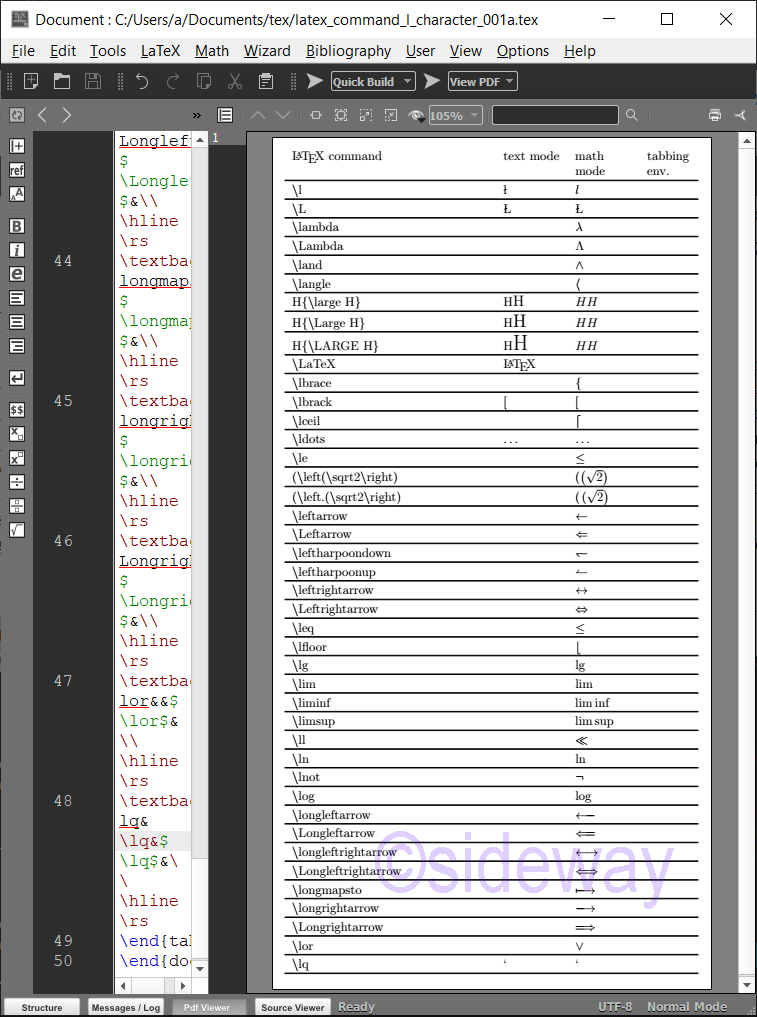output.to from Sideway
Draft for Information Only

# Content

LaTeX \l⋯ Commands for Printing Characters \l⋯ Commands \l⋯ Command Examples Source and Reference

# LaTeX \l⋯ Commands for Printing Characters

LaTeX uses backslash+l⋯ combination to print characters.

## \l⋯ Commands

The typical \l⋯ Commands for Printing Characters are LaTeX CommandsRemarksMode \lprints a Latin small Letter L with Stroke, ł. \Lprints a Latin capital letter L with stroke, Ł. \lambdaprints a Greek small letter lamda, λ.math mode \Lambdaprints a Greek capital letter lamda, Λ.math mode \landprints a logical and, ∧.math mode \langleprints a mathematical left angle bracket, ⟨.math mode \largeprints a large typeface. e.g. HH \Largeprints a very large typeface. e.g. HH \LARGEprints a very very large typeface. e.g. HH \LaTeXprints the LaTeX logo. \lbraceprints a left curly bracket or brace, {.math mode \lbrackprints a left square bracket or bracket, [.math mode \lceilprints a left ceiling, ⌈.math mode \ldotsprints a horizontal ellipsis ,…. \leprints a less-than or equal to, ≤.math mode \leftprints a dynamic delimiter depending on the content.math mode \left.prints a null delimiter for pairing with \right.math mode \leftarrowprints a leftwards arrow, ←.math mode \Leftarrowprints a leftwards double arrow, ⇐.math mode \leftharpoondownprints a leftwards harpoon with barb downwards, ↽.math mode \leftharpoonupprints a leftwards harpoon with barb upwards, ↼.math mode \leftrightarrowprints a left right arrow, ↔.math mode \Leftrightarrowprints a left right double arrow, ⇔.math mode \leqprints a less-than or equal to, ≤.math mode \lfloorprints a left floor, ⌊.math mode \lgprints math, lg.math mode \limprints math, lim.math mode \liminfprints math, lim inf.math mode \limsupprints math, lim sup.math mode \llprints a much less-than, ≪.math mode \lnprints math, ln.math mode \lnotprints a not sign, ¬.math mode \logprints math, log.math mode \longleftarrowprints a long leftwards arrow, ⟵.math mode \Longleftarrowprints a long leftwards double arrow, ⟸.math mode \longleftrightarrowprints a long left right arrow, ⟷.math mode \Longleftrightarrowprints a long left right double arrow, ⟺.math mode \longmapstoprints a long rightwards arrow from bar, ⟼.math mode \longrightarrowprints a long rightwards arrow, ⟶.math mode \Longrightarrowprints a long rightwards double arrow, ⟹.math mode \lorprints a logical or, ∨.math mode \lqprints a left single quotation mark, ‘.

## \l⋯ Command Examples

Examples of LaTeX commands
###### Code
\documentclass[border=10pt,varwidth]{standalone}
\newcommand\rs{\hline\noalign{\vskip 0.2em}}
\newenvironment{tabtest} {\setlength{\topsep}{0mm}\setlength{\partopsep}{0mm}\begin{tabbing}\hspace*{9mm}\kill}{\end{tabbing} }
\begin{document}
\begin{tabular}{p{0.45\textwidth}p{.13\textwidth}p{.13\textwidth}p{.1\textwidth}}
\LaTeX\ command & text mode & math mode & tabbing env.\\\hline\rs
\textbackslash l&\l&$\l$&\\\hline\rs
\textbackslash L&\L&$\L$&\\\hline\rs
\textbackslash lambda&&$\lambda$&\\\hline\rs
\textbackslash Lambda&&$\Lambda$&\\\hline\rs
\textbackslash land&&$\land$&\\\hline\rs
\textbackslash langle&&$\langle$&\\\hline\rs
H\{\textbackslash large H\}&H{\large H}&$H{\large H}$&\\\hline\rs
H\{\textbackslash Large H\}&H{\Large H}&$H{\Large H}$&\\\hline\rs
H\{\textbackslash LARGE H\}&H{\LARGE H}&$H{\LARGE H}$&\\\hline\rs
\textbackslash LaTeX&\LaTeX&&\\\hline\rs
\textbackslash lbrace&&$\lbrace$&\\\hline\rs
\textbackslash lbrack&\lbrack&$\lbrack$&\\\hline\rs
\textbackslash lceil&&$\lceil$&\\\hline\rs
\textbackslash ldots&\ldots&$\ldots$&\\\hline\rs
\textbackslash le&&$\le$&\\\hline\rs
(\textbackslash left(\textbackslash sqrt2\textbackslash right)&&$(\left(\sqrt2\right)$&\\\hline\rs
(\textbackslash left.(\textbackslash sqrt2\textbackslash right)&&$(\left.(\sqrt2\right)$&\\\hline\rs
\textbackslash leftarrow&&$\leftarrow$&\\\hline\rs
\textbackslash Leftarrow&&$\Leftarrow$&\\\hline\rs
\textbackslash leftharpoondown&&$\leftharpoondown$&\\\hline\rs
\textbackslash leftharpoonup&&$\leftharpoonup$&\\\hline\rs
\textbackslash leftrightarrow&&$\leftrightarrow$&\\\hline\rs
\textbackslash Leftrightarrow&&$\Leftrightarrow$&\\\hline\rs
\textbackslash leq&&$\leq$&\\\hline\rs
\textbackslash lfloor&&$\lfloor$&\\\hline\rs
\textbackslash lg&&$\lg$&\\\hline\rs
\textbackslash lim&&$\lim$&\\\hline\rs
\textbackslash liminf&&$\liminf$&\\\hline\rs
\textbackslash limsup&&$\limsup$&\\\hline\rs
\textbackslash ll&&$\ll$&\\\hline\rs
\textbackslash ln&&$\ln$&\\\hline\rs
\textbackslash lnot&&$\lnot$&\\\hline\rs
\textbackslash log&&$\log$&\\\hline\rs
\textbackslash longleftarrow&&$\longleftarrow$&\\\hline\rs
\textbackslash Longleftarrow&&$\Longleftarrow$&\\\hline\rs
\textbackslash longleftrightarrow&&$\longleftrightarrow$&\\\hline\rs
\textbackslash Longleftrightarrow&&$\Longleftrightarrow$&\\\hline\rs
\textbackslash longmapsto&&$\longmapsto$&\\\hline\rs
\textbackslash longrightarrow&&$\longrightarrow$&\\\hline\rs
\textbackslash Longrightarrow&&$\Longrightarrow$&\\\hline\rs
\textbackslash lor&&$\lor$&\\\hline\rs
\textbackslash lq&\lq&$\lq$&\\\hline\rs
\end{tabular}
\end{document}
###### Output## Source and Reference

©sideway

ID: 210200026 Last Updated: 2/26/2021 Revision: 0Home 5

Business

Management

HBR 3

Information

Recreation

Culture

Chinese 1097

English 337

Computer

Hardware 153

Software

Application 204

Latex 34

Manim 171

Numeric 19

Programming

Web 285

Unicode 504

HTML 65

CSS 59

ASP.NET 194

OS 409

Python 56

Knowledge

Mathematics

Algebra 30

Geometry 21

Calculus 67

Engineering

Mechanical

Rigid Bodies

Statics 92

Dynamics 37

Control

Natural Sciences

Electric 27

Copyright © 2000-2021 Sideway . All rights reserved Disclaimers last modified on 06 September 2019